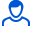Content: 00100.png (38.19 KB)

Positive responses: 0
Negative responses: 0

Sold: 0
Refunds: 0Loyalty discount! If the total amount of your purchases from the seller more than:

 \$1 the discount is 10% \$5 the discount is 30%

In ultracentrifuges, the rate of displacement of the molecules of the studied polymer in the direction from the axis of rotation is expressed by the formula V = bω2t, where b is a constant; ω is the angular velocity of the centrifuge; x is the distance from the axis of rotation to the moving boundary of the settling polymer. Determine the equation of motion of the polymer boundary if at time t = 0 it was at a distance of 0.5 cm from the axis of rotation.
Task 00100. Detailed solution with a brief record of the conditions, formulas and laws used in the decision, the conclusion of the calculation formula and the answer.
If you have any questions about the decision, write. I will try to help. File in image format.
No feedback yet